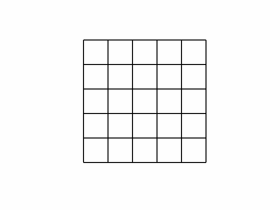# Magic Squares, Part 2, AlgorithmsThe magic squares of odd order generated by MATLAB show a pattern with increasing elements generally moving diagonally up and to the right.

### Contents

#### Three Cases

The algorithms used by MATLAB for generating magic squares of order n fall into three cases:
• odd, n is odd.
• doubly-even, n is divisible by 4.
• singly-even, n is divisible by 2, but not by 4.

#### Odd Order

The best known algorithm for generating magic squares of odd order is de La Loubere's method. Simon de La Loubere was the French ambassador to Siam in the late 17th century. I sometimes refer to his method as the "nor'easter algorithm", after the winter storms that move northeasterly up the coast of New England. You can see why if you follow the integers sequentially through magic(9).
   47    58    69    80     1    12    23    34    45
57    68    79     9    11    22    33    44    46
67    78     8    10    21    32    43    54    56
77     7    18    20    31    42    53    55    66
6    17    19    30    41    52    63    65    76
16    27    29    40    51    62    64    75     5
26    28    39    50    61    72    74     4    15
36    38    49    60    71    73     3    14    25
37    48    59    70    81     2    13    24    35
The integers from $1$ to $n^2$ are inserted along diagonals, starting in the middle of first row and heading in a northeasterly direction. When you go off an edge of the array, which you do at the very first step, continue from the opposite edge. When you bump into a cell that is already occupied, drop down one row and continue. We used this algorithm in MATLAB for many years. Here is the code.
   A = zeros(n,n);
i = 1;
j = (n+1)/2;
for k = 1:n^2
is = i;
js = j;
A(i,j) = k;
i = n - rem(n+1-i,n);
j = rem(j,n) + 1;
if A(i,j) ~= 0
i = rem(is,n) + 1;
j = js;
end
end
A big difficulty with this algorithm and resulting program is that it inserts the elements one at a time---it cannot be vectorized.

#### A New Algorithm

A few years ago we discovered an algorithm for generating the same magic squares of odd order as de La Loubere's method, but with just four MATLAB matrix operations.
   [I,J] = ndgrid(1:n);
A = mod(I+J+(n-3)/2,n);
B = mod(I+2*J-2,n);
M = n*A + B + 1;
Let's see how this works with n = 5. The statement
   [I,J] = ndgrid(1:n)
produces a pair of matrices whose elements are just the row and column indices, i and j.
   I =
1     1     1     1     1
2     2     2     2     2
3     3     3     3     3
4     4     4     4     4
5     5     5     5     5
   J =
1     2     3     4     5
1     2     3     4     5
1     2     3     4     5
1     2     3     4     5
1     2     3     4     5
Using these indices, we generate two more matrices. The statements
   A = mod(I+J+1,n)
B = mod(I+2*J-2,n)
produce
   A =
3     4     0     1     2
4     0     1     2     3
0     1     2     3     4
1     2     3     4     0
2     3     4     0     1
   B =
1     3     0     2     4
2     4     1     3     0
3     0     2     4     1
4     1     3     0     2
0     2     4     1     3
Both A and B are fledgling magic squares. They have equal row, column and diagonal sums. But their elements are not the integers from $1$ to $n^2$. Each has duplicated elements between $0$ and $n-1$. The final statement
   M = n*A+B+1
produces a matrix whose elements are integers between $1$ and $n^2$ and which has equal row, column and diagonal sums. What is not obvious, but is true, is that there are no duplicates. So M must contain all of the integers between $1$ and $n^2$ and consequently is a magic square.
   M =
17    24     1     8    15
23     5     7    14    16
4     6    13    20    22
10    12    19    21     3
11    18    25     2     9

#### Doubly Even Order

The doubly-even algorithm is also short and sweet, and tricky.
   M = reshape(1:n^2,n,n)';
[I,J] = ndgrid(1:n);
K = fix(mod(I,4)/2) == fix(mod(J,4)/2);
M(K) = n^2+1 - M(K);
Let's look at our friend magic(4). The matrix M is initially just the integers from 1 to 16 stored sequentially in 4 rows.
   M =
1     2     3     4
5     6     7     8
9    10    11    12
13    14    15    16
The logical array K is true for half of the indices and false for the other half in a pattern like this.
   K =
1     0     0     1
0     1     1     0
0     1     1     0
1     0     0     1
The elements where K is false, that is 0, are left alone.
        .     2     3     .
5     .     .     8
9     .     .    12
.    14    15     .
The elements where K is true, that is 1, are reversed.
       16     .     .    13
.    11    10     .
.     7     6     .
4     .     .     1
The final result merges these two matrices to produce the magic square.

#### Singly Even Order

The algorithm for singly even order is the most complicated and so we will give just a glimpse of how it works. If n is singly even, then $n/2$ is odd and magic(n) can be constructed from four copies of magic(n/2). For example, magic(10) is obtained from A = magic(5) by forming a block matrix.
   [  A      A+50
A+75    A+25 ]
The column sums are all equal because sum(A) + sum(A+75) equals sum(A+50) + sum(A+25). But the rows sums are not quite right. The algorithm must finish by doing a few swaps of pieces of rows to clean up the row sums. For the details, issue the command.
   type magic